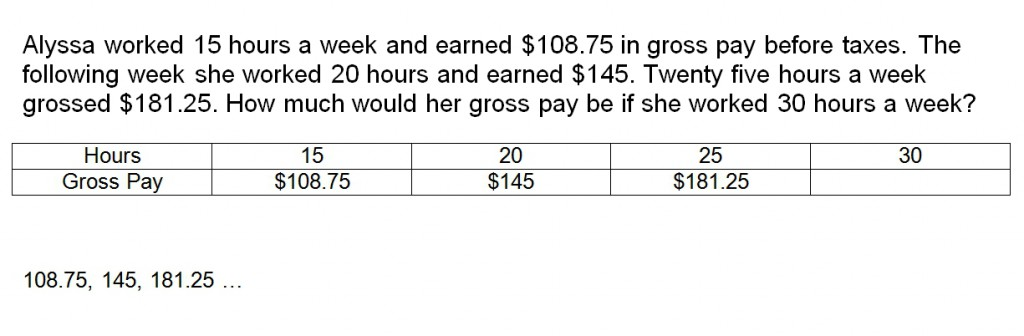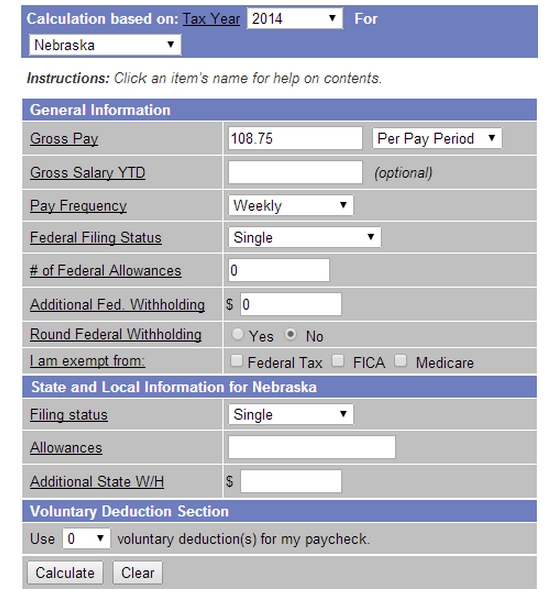# Patterns

//Patterns
Patterns 2017-11-13T22:01:53+00:00

Stacie Bender 8/14/14

Numbers often follow patterns in real life. Sometimes adding the same number over and over creates a series. Other patterns are more complex.

## Example 1

Let’s look at the measurements of the angles of some polygons. Notice that the sum of the interior angles of these figures is not only constant, but follows a pattern. First let’s figure out what the pattern is.If we take the quadrilaterals’ sum of 360 and subtract the triangles sum of 180 we get 180°. 540 for the pentagon minus 360 for the quadrilateral again gets us 180° Now, can you find the sum of the interior angles of a hexagon? Add the pentagon’s value to 180. How would you find the sum of the interior angles of an octagon? Add 180 twice to the hexagon’s value.

## Example 2

Alyssa worked 15 hours a week and earned \$108.75 in gross pay before taxes. The following week she worked 20 hours and earned \$145. Twenty five hours a week grossed \$181.25. How much would her gross pay be if she worked 30 hours a week? Don’t shy away because this is a story problem. To visualize it, we can create a table as we did in the previous example.Because the hours are increasing by a constant rate, and the gross pay is requested for the next incremented value, the pattern can also be written simply as 108.75, 145, 181.25… The three docs known as an ellipsis indicate that the pattern continues. In this real world example, the pattern would be accurate for values between 0 and 40 hours. Why would the pattern likely change above 40 hours?

## Example 3

Steve Jobs coined the phrase “put 1000 songs in your pocket” with the initial release of the iPod. Since then storage capacities and the estimated number of songs that would fit in iPods has grown. Do they follow a pattern?http://support.apple.com/kb/SP99 If you are curious why the estimates of the first two generations don’t fit the rest of the pattern, research the difference between MP3 and AAC encoding.

## Investigate Further

Use the link below to estimate the take-home pay for 15, 20, 25, and 30 hours of work in your state. http://www.adp.com/tools-and-resources/calculators-and-tools/payroll-calculators/salary-paycheck-calculator.aspxText:

Holt, Rinehart and Winston Algebra 1 p. 4 – 10